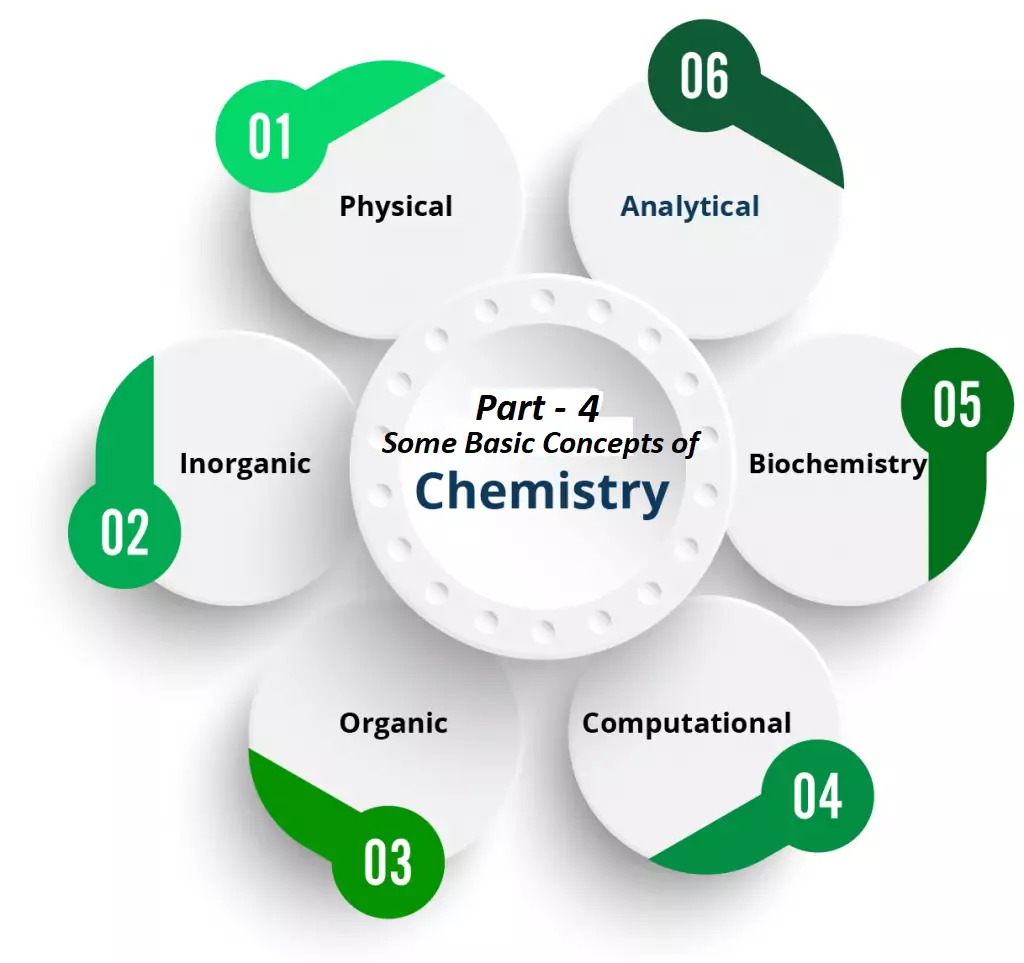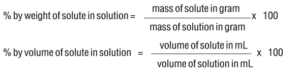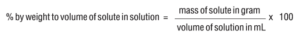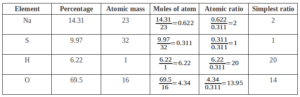# Empirical and Molecular formula And Limiting ReagentEmpirical and Molecular formula And Limiting Reagent

Percentage composition: It is the percentage of each element present in 100g of a substance.
Percentage composition (mass percent) of an element
= Mass of that element in the compound x 100
The molar mass of the compoundEmpirical Formula: It represents the simplest whole-number ratio of various atoms present in a compound. e.g., CH is the empirical formula of benzene (C6H6).

Molecular Formula: It shows the exact number of different atoms present in a molecule of a compound. e.g., C6H6 is the molecular formula of benzene.

Relationship between empirical and molecular formulae:
Molecular formula = n × Empirical formula
Where; n = Molecular Mass
Empirical Formula Mass

The following Steps Should be Followed to Determine the Empirical Formula of the Compound:
Step 1: Conversion of mass percent of various elements into grams.
Step 2: Convert mass obtained into the number of moles.

Empirical and Molecular formula And Limiting Reagent

Step 3: Divide the mole value obtained by the smallest mole value (out of the mole value of various elements calculated)
Step 4: If the ratios are not whole numbers, then they may be converted into a whole number by multiplying with the suitable coefficient.
Step 5: Write the empirical formula by mentioning the numbers after writing the symbols of the respective elements.

The Post Empirical and Molecular formula And Limiting Reagent are very important to understand the basic concept let’s take an example:

Que. A compound on analysis gave the following percentage composition Na=14.31% S=9.97% H=6.22% O=69.5%. Calculate the molecular formula of the compound on the assumption that all hydrogen in the compound present in the combination with oxygen as the water of crystallization. The molecular mass of the compound is 322. Na=23 S=32 O=16 H=1.

Ans.Empirical Formula = Na2SH20O14
We Know
Molecular formula = n × Empirical formula
Where; n = Molecular Mass
Empirical Formula Mass
n = 322/322 = 1
Molecular Formula = 1 × Na2SH20O20 = Na2SH20O20
Final Molecular Formula – Na2SO4.10H2O

Limiting reagent (Limiting reactant): The reagent which limits a reaction or the reagent which is completely consumed in a chemical reaction is called limiting reagent or limiting reactant.
For e.g. in the reaction
2SO2(g) + O2(g)    2 SO3(g), 2 moles of SO2 reacts completely with 1 mole of O2 to form 2 moles of SO3. If we take 10 moles each of SO2 and O2, we get only 10 moles of SO3 because 10 moles of SO2 require only 5 moles of O2 for the complete reaction. So here SO2 is the limiting reagent and 5 moles of O2 remains unreacted.

Reactions in solutions:
Solutions are homogeneous mixture containing 2 or more components. The component which is present in larger quantity is called Solvent and the other components are called Solutes. Or, the substance which is dissolved is called the solute, and the substance in which solute is dissolved is called the solvent. For e.g. in NaCl solution, NaCl is the solute and water is the solvent. A solution containing only 2 components are called Binary Solution. If the solvent is water, it is called an aqueous solution.

Empirical and Molecular formula And Limiting Reagent

SOME BASIC CONCEPTS OF CHEMISTRY – 1

Some Basic Concepts of Chemistry Part – 2

Some Basic Concepts of Chemistry Mole Concept Part – 3